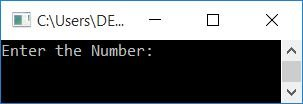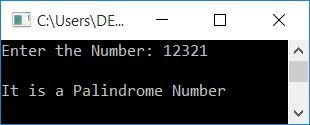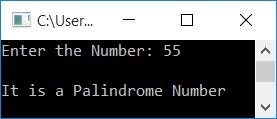# C++ program to check palindrome numbers

In this article, you will learn and get code to check whether a number entered by the user (at run-time) is a palindrome number or not in C++. Here is the list of programs for checking palindrome numbers:

### What is a palindrome number?

If the inverse of a number is equal to the number itself, then it is called a palindrome number. For example, 121, 15751, etc.

## Check if a number is a palindrome without using a function

To check whether a number given by the user is a palindrome number or not in C++, first receive the number, initialize its value to a variable, say temp, and reverse the value of temp. After reversing, check whether the original number is equal to temp or not.

That is, if the original number is equal to the value of rev (the original number's reverse or temp's reverse), then print a message like, it is a palindrome number, otherwise print a message like, it is not a palindrome number, as shown in the program given below:

```#include<iostream>
using namespace std;
int main()
{
int num, rev=0, rem, temp;
cout<<"Enter the Number: ";
cin>>num;
temp = num;
while(temp>0)
{
rem = temp%10;
rev = (rev*10)+rem;
temp = temp/10;
}
if(rev==num)
cout<<"\nIt is a Palindrome Number";
else
cout<<"\nIt is not a Palindrome Number";
cout<<endl;
return 0;
}```

This program was built and runs under the Code::Blocks IDE. Here is its sample run:Now supply the input, say 12321, and press the ENTER key to check and print whether it is a palindrome number or not, as shown in the snapshot given below:Here is another sample run with user input, 12345:In the above program, we have initialized the original number stored in num to temp. The value of temp is then reversed. Finally, we compared the reversed value (value of rev) to the original number (value of num). If it is found to be equal, then print it as a palindrome number; otherwise, print it as a non-palindrome number.

To learn more about reversing a number, refer to C++ Program to Reverse a Number article. It contains all of the necessary information.

## Check if a number is a palindrome number using a function

The question is: write a program in C++ that checks for palindromes or not using a user-defined function? Here is its answer:

```#include<iostream>
using namespace std;
int checkPalindrome(int);
int main()
{
int num, val;
cout<<"Enter the Number: ";
cin>>num;
val = checkPalindrome(num);
if(val==0)
cout<<"\nIt is a Palindrome Number";
else
cout<<"\nIt is not a Palindrome Number";
cout<<endl;
return 0;
}
int checkPalindrome(int n)
{
int temp, rem, rev=0;
temp = n;
while(temp>0)
{
rem = temp%10;
rev = (rev*10)+rem;
temp = temp/10;
}
if(rev==n)
return 0;
else
return 1;
}```

Here is its sample run with user input, 55:#### The same program in different languages

C++ Quiz

« Previous Program Next Program »